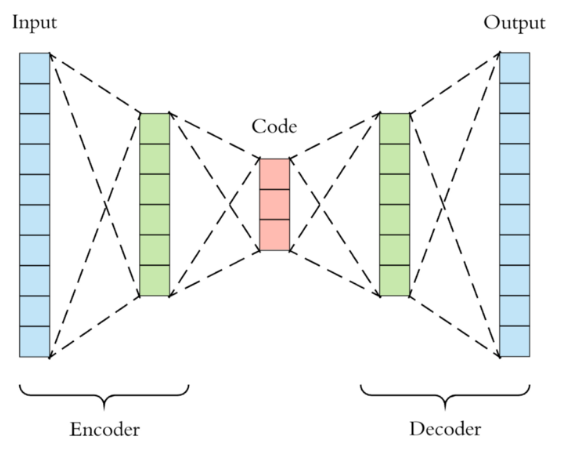Audio autoencoder error: Trying to create tensor with negative dimension

Hii, I’m trying to create an Audio Autoencoder using pytorch with 3 layers, the goal is to be something like this:But I’m getting this error message:

RuntimeError: Trying to create tensor with negative dimension -1007: [512, 1, 1, -1007]

Here is my model class, I used a yt video as example since I’m very noob with PyTorch. Does anyone know what am I doing wrong?class Convautoenc(nn.Module):
def __init__(self):
super(Convautoenc, self).__init__()

self.bn1 =nn.BatchNorm1d(16)
self.drop=nn.Dropout()
self.max_pool=nn.MaxPool1d(kernel_size=2,stride=2)
self.mid1=nn.Linear(12511,16,bias=True)

self.synconv1=nn.ConvTranspose1d(in_channels=16, out_channels=1, kernel_size=1024, stride=1, padding=1023, bias=True)

def encoder(self, x):
#Analysis:
x = self.conv1(x)
y = torch.tanh(x)
return x

def middle(self, z):
z = self.max_pool(z)
z = self.drop(z)
z = self.mid1(z)
z = self.drop(z)
return z

def decoder(self, y):
#Synthesis:
xrek= self.synconv1(y)
return xrek

def forward(self, x):
y=self.encoder(x)
z=self.middle(y)
#y=torch.round(y/0.125)*0.125
xrek=self.decoder(z)
return xrek

Your model architecture doesn’t match the posted image, which seems to use linear layers only.
In your current model you are mixing nn.Conv1d layers (which expect 3D inputs as [batch_size, channels, seq_len]) with nn.Linear layers (which accept [batch_size, *, in_feautres] inputs).
self.mid will create an output as [batch_size, *, 16] while the following self.syncconv1 layer would try to apply a kernel with a size of 1024 to the sequence length of 16 and fails with the negative output size.

Hi @ptrblck , thank you for you awnser!
Before I tried to add this middle layer, the autoencoder was working only with the Conv1d and ConvTranspose1d. But since I need this middle one with a small size for my project, I was told by a friend that I should add a Linear layer.
After reading your awnser, I’m not sure whether this is a good approach or if I’m just being stupidDo you know, if there is a way to pass the Linear layer output as an input for the Transpose1D?

Adding a linear layer might work, but you would need to check how it should be used on its inputs.
As described before, the incoming activation is seen as [batch_size, channels, seq_len] and the linear layer accepts [batch_size, *, nb_features], where the * denotes additional dimensions.
In your case the linear layer will be applied on each channel separately and will thus yield an output of [batch_size, channels, out_features].
This operation would thus transform the temporal dimension to the out_features. I’m not familiar with your use case but you should check if this is indeed what you want.
On the other hand, if you want to keep the temporal dimension and apply the linear layer on each time step, permute the input activation to [batch_size, seq_len, channels] and permute it back before feeding it into the transposed conv layer.

Hii, after some tests, I found out that using a Linear layer to deal with audio signals is a bad ideia because it kinda loses the time reference? I don’t quite understand the mathematics behind, but all my tests and models points towards that.
I manage insert 2 middle layers that still reduces the size of the model, using 1 extra conv1D and 1 convTranspose1d.
I will leave my final model here for it might help other people facing the same issue.
Thank you for all the explanations!!

class Convautoenc(nn.Module):
def __init__(self):
super(Convautoenc, self).__init__()
self.conv1=nn.Conv1d(in_channels=1, out_channels=16, kernel_size=1024, stride=1, padding=1023, bias=True)
self.conv2=nn.Conv1d(in_channels=16, out_channels=32, kernel_size=512, stride=8, padding=1, bias=True)
self.synconv2=nn.ConvTranspose1d(in_channels=32, out_channels=16, kernel_size=512, stride=8, padding=1, bias=True)
self.synconv1=nn.ConvTranspose1d(in_channels=16, out_channels=1, kernel_size=1023, stride=1, padding=1022, bias=True)

def encoder(self, x):
x = self.conv1(x)
x = torch.tanh(x)
x = self.conv2(x)
return x

def decoder(self, y):
y = self.synconv2(y)
y = self.synconv1(y)
return y

def forward(self, x):
y=self.encoder(x)
y=self.decoder(y)
return y